[子集] 假定AB都是集,B的每个元素都是A的元素,那末称BA的一个子集,记作B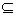AA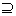B.“”读作“包含于”或“被掩于”，“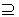”读作“包含”或“掩盖”.

对于任何的集A,BC

AA                        (自反律)

AB,BA,可推出A=B   (反对称律)

AB,BC,则AC        (传递律)

假定BA但是B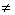A(B=A不成立),那末称BA的一个真子集,记作(B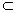A).

规定空集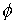是任何集的子集.

[变进的变换] 假定一个变换f把一个集X变上集Y的一个子集,那末称f为把X变进Y的变换,简称f是变进的(映入的).变上是变进的特殊情况.

[划分公理与特征函数] 假定有一个变换f把一个集X变进{0,1},那末1的所有象源的全体是X的一个子集X',f称为X'的特征函数.

划分公理是替换公理的结论,因为如果1的象源全体是φ,那末φ当然是X的子集,否则1至少有一个象源x0X,造一个变换

`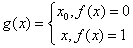`

推论  假定X是集,对每个x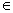X,论点p(x)和­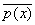(p(x)的否定)一定有一个且只有一个成立,那末{x|xXp(x)}是一个集.

[差集与余集] 假定AB都是集,那末所有属于A但不属于B的元素的全体是一个集(由划分公理的推论),称为AB的差集记作A\B.

特别,当BA的时候,A\B称为BA中的余集.

[方幂集公理] 一个集A的所有子集的全体是一个集,记作,称为A方幂集.

可以把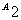一对一地变上“所有把A变进2 = {01}的变换的全体”去，所以后者也是一个集，这个集和A方幂集可以互相作为彼此的标号集.今后,往往把它们看作同一个集,也就是把A的一个子集跟它的一个特征函数混同起来.

[和集(并)与和集公理] 假定{Ah|hH}是一个集族,那末{x|存在一个Ah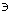x}是一个集,它称为这族集的和集(并),记作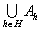.

当一个集族的全部集是A,B,C,…时,这族集的和集可写成

ABC

{1,2,3}∪{0,2,4}∪{2,1}={0,1,2,3,4}

[通集(交)] 假定{Ah|hH}是一个集族,那末{x|所有的Ahx}是一个集,它称为这族集的通集(交),记作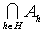.通集存在是划分公理的结论.

当一个集族的全部集是A,B,C,…时,这族集的通集可写成

ABC

{1,2,3}∩{0,2,4}∩{2,1}={2}

[直接积(笛卡儿积)] 假定A={xh|hH},B={yk|kK},那末

{<xh,yk>|xhAykB}

直接积存在是替换公理与和集公理的结论.因为对任何hHkK,{<xh,yk>}是一个集,由替换公理,{{<xh,yk>}|hH}是一个集族,因此存在和集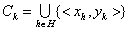.{Ck|kK}又是一个集族,所以又存在和集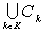,这就是A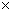B.

假定{Ah|hH }是一个集族,其中每个Ah ¹φ那末由选择公理(§2,一)对每个hH可以得到一个xhAh,并且由替换公理得到一个集

<xh|hH>={{ xh ,h}|hH}

把每个xhAh 换为一个x'hAh ,那末由替换公理得到另一个集

<x'h|hH>={{ x'h ,h}|hH}

所有这种有序组的全体是一个集，它称为一族集Ah(hH)的直接积,记作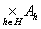.

H=2,就是AB.

[叠集] 假定AB都是集,那末由变换的定义,每个把A变进B的变换f都是AB的子集,因此f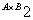.由划分公理,所有把A变进B的变换f的全体{f|ffA变进B}是一个集,称为把A叠在B上的叠集,记作AB.

显然, AB.另一方面,特别当B=2={0,1}时,A2既是方幂集又是叠集.

[集的运算规律]A,B,C都是集,则

交换律        AB=BA,AB=BA

结合律        A(BC)=(ΑB)C

A(ΒC)=(ΑB)C

分配律        A(BC)=(AB)(AC)

A(BC)=(AB)(AC)

×摩根(De Morgan)律

C\(AB)=(C\A)(C\B)

C\(AB)=(C\A)(C\B)